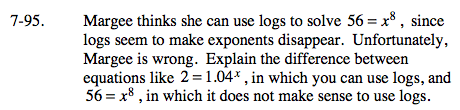Home > A2C > Chapter 7 > Lesson 7.2.1 > Problem7-95

7-95.

Margee thinks she can use logs to solve 56 = x8, since logs seem to make exponents disappear. Unfortunately, Margee is wrong. Explain the difference between equations like 2 = 1.04x , in which you can use logs, and 56 = x8, in which it does not make sense to use logs. Homework Help ✎Look at where the variable is in each equation.

Logarithms are used to solve equations where the variable is the exponent.
Roots (or raising to a fractional exponent) are used to solve equations where
the exponent is known and the variable is the base.

$\text{Margee should raise both sides of the equation to the } \frac{1}{8}\text{th power.}$